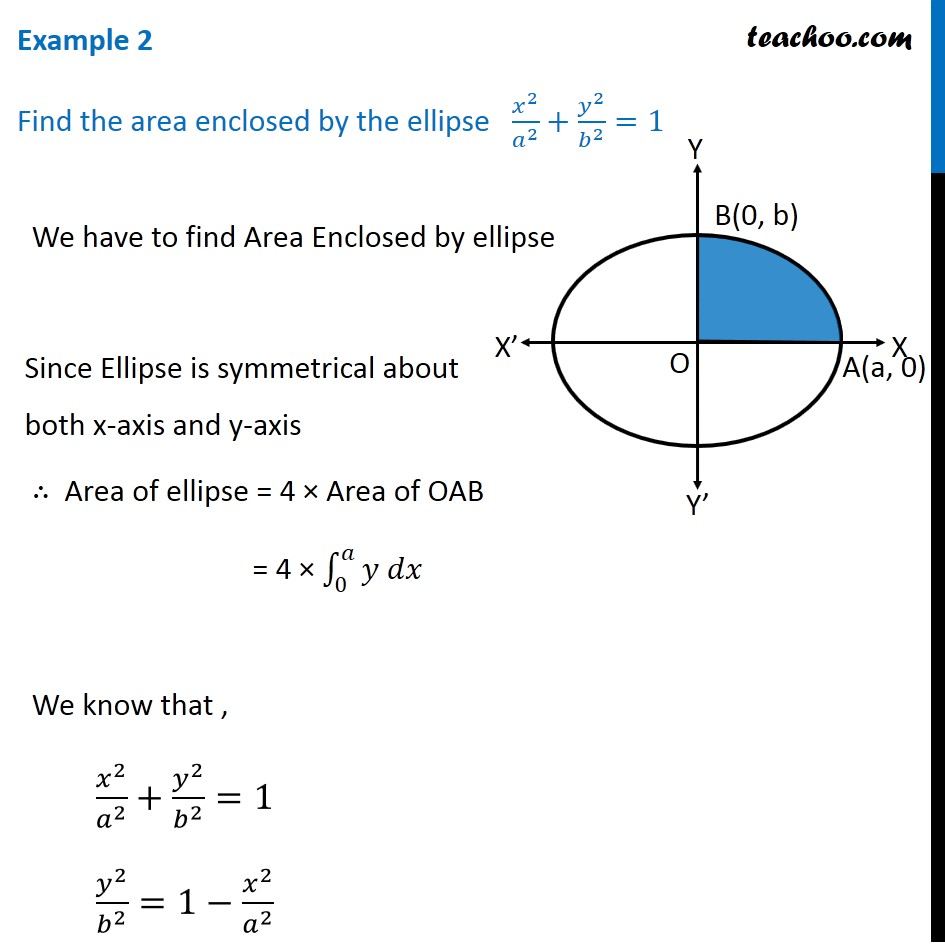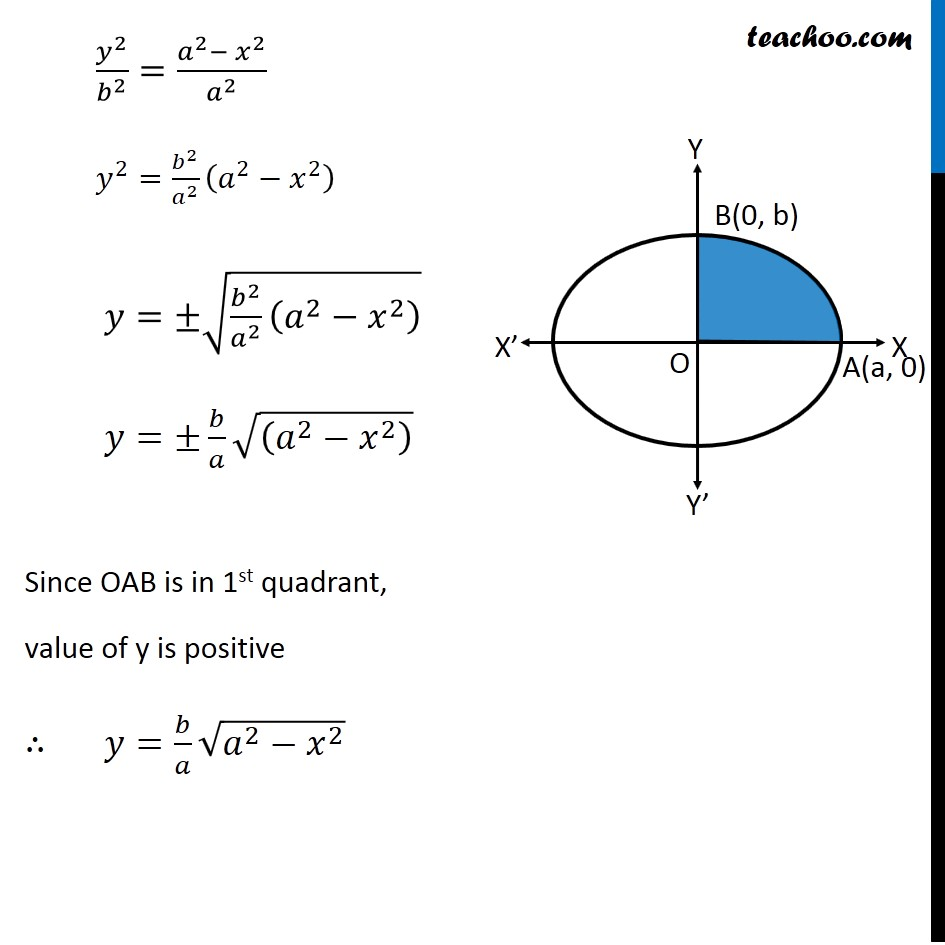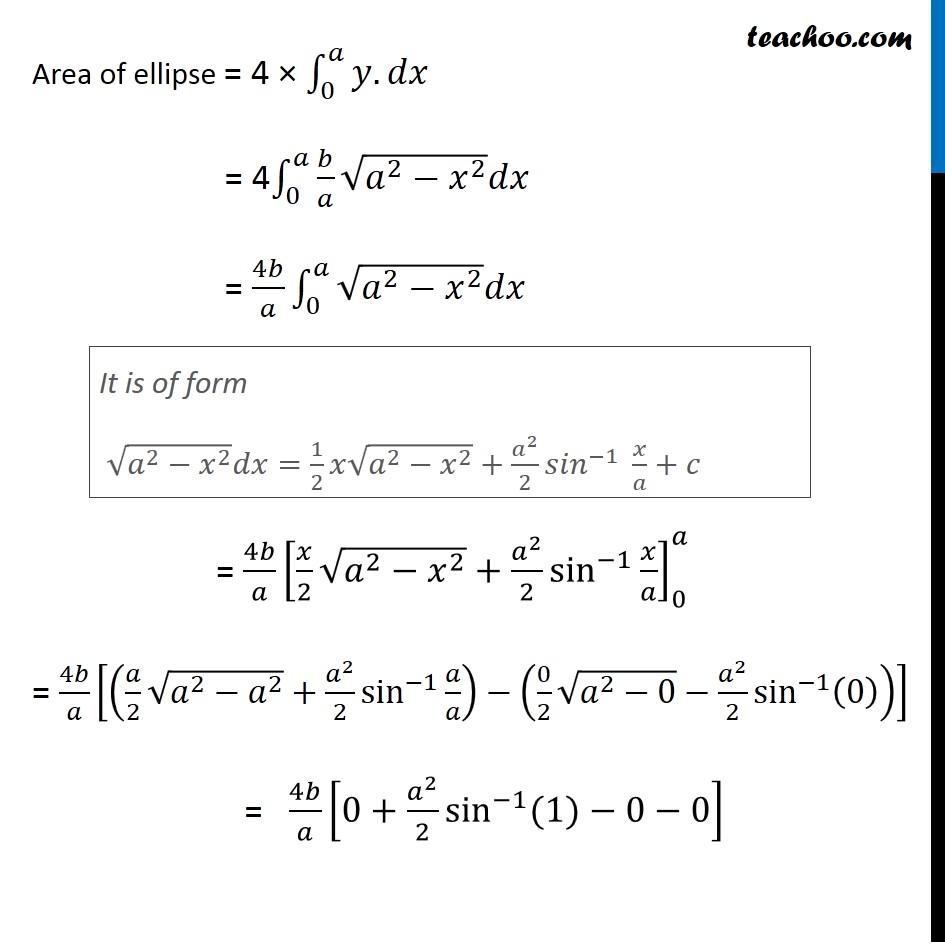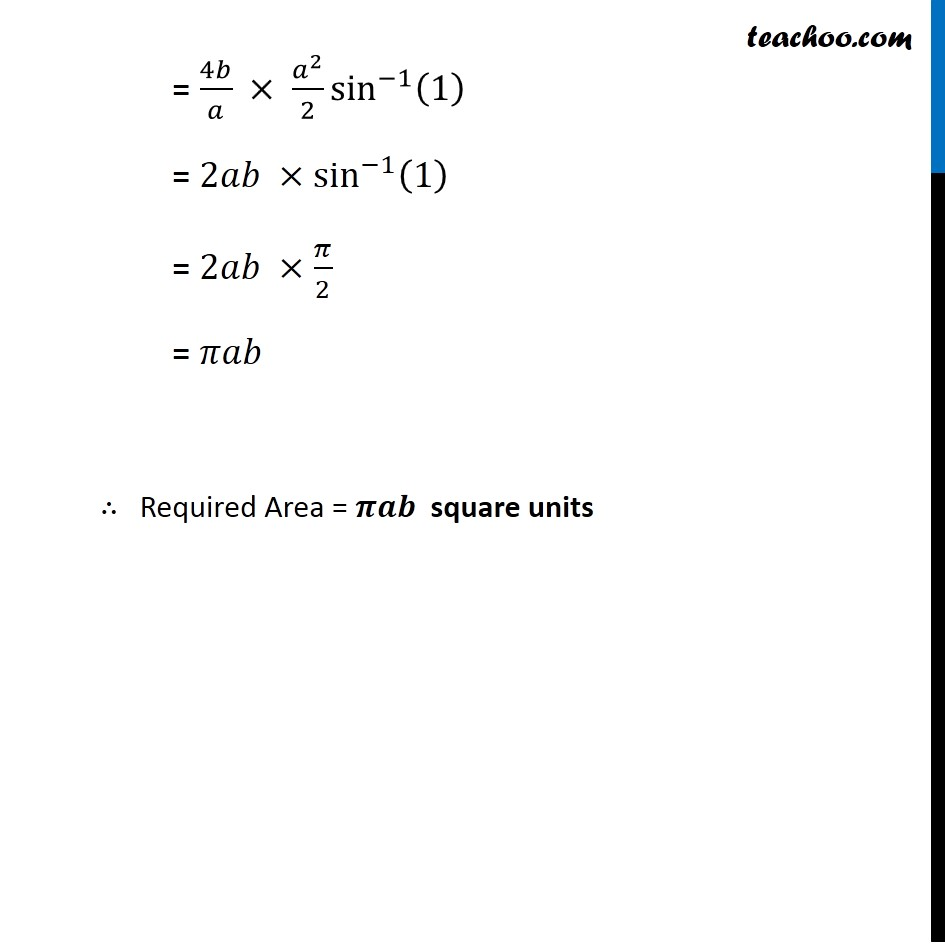Area under curve

Chapter 8 Class 12 Application of Integrals
Concept wiseIntroducing your new favourite teacher - Teachoo Black, at only ₹83 per month

### Transcript

Example 2 Find the area enclosed by the ellipse 𝑥^2/𝑎^2 +𝑦^2/𝑏^2 =1 We have to find Area Enclosed by ellipse Since Ellipse is symmetrical about both x-axis and y-axis ∴ Area of ellipse = 4 × Area of OAB = 4 × ∫_0^𝑎▒〖𝑦 𝑑𝑥〗 We know that , 𝑥^2/𝑎^2 +𝑦^2/𝑏^2 =1 𝑦^2/𝑏^2 =1−𝑥^2/𝑎^2 𝑦^2/𝑏^2 =(𝑎^2−𝑥^2)/𝑎^2 𝑦^2=𝑏^2/𝑎^2 (𝑎^2−𝑥^2 ) ∴ 𝑦=±√(𝑏^2/𝑎^2 (𝑎^2−𝑥^2 ) ) 𝑦=±𝑏/𝑎 √((𝑎^2−𝑥^2 ) ) Since OAB is in 1st quadrant, value of y is positive ∴ 𝑦=𝑏/𝑎 √(𝑎^2−𝑥^2 ) Area of ellipse = 4 × ∫_0^𝑎▒〖𝑦.𝑑𝑥〗 = 4∫_0^𝑎▒𝑏/𝑎 √(𝑎^2−𝑥^2 ) 𝑑𝑥 = 4𝑏/𝑎 ∫_0^𝑎▒〖√(𝑎^2−𝑥^2 ) 𝑑𝑥" " 〗 = 4𝑏/𝑎 [𝑥/2 √(𝑎^2−𝑥^2 )+𝑎^2/2 sin^(−1)⁡〖𝑥/𝑎〗 ]_0^𝑎 = 4𝑏/𝑎 [(𝑎/2 √(𝑎^2−𝑎^2 )+𝑎^2/2 sin^(−1)⁡〖𝑎/𝑎〗 )−(0/2 √(𝑎^2−0)−𝑎^2/2 sin^(−1)⁡(0) )] = 4𝑏/𝑎 [0+𝑎^2/2 sin^(−1)⁡〖(1)〗−0−0] It is of form √(𝑎^2−𝑥^2 ) 𝑑𝑥=1/2 𝑥√(𝑎^2−𝑥^2 )+𝑎^2/2 〖𝑠𝑖𝑛〗^(−1)⁡〖 𝑥/𝑎+𝑐〗 = 4𝑏/𝑎 × 𝑎^2/2 sin^(−1)⁡(1) = 2𝑎𝑏 ×sin^(−1)⁡(1) = 2𝑎𝑏 × 𝜋/2 = 𝜋𝑎𝑏 ∴ Required Area = 𝝅𝒂𝒃 square units